Test: Power System Stability of Power Systems

# Test: Power System Stability of Power Systems

Test Description

## 10 Questions MCQ Test GATE Electrical Engineering (EE) 2023 Mock Test Series | Test: Power System Stability of Power Systems

Test: Power System Stability of Power Systems for Electrical Engineering (EE) 2023 is part of GATE Electrical Engineering (EE) 2023 Mock Test Series preparation. The Test: Power System Stability of Power Systems questions and answers have been prepared according to the Electrical Engineering (EE) exam syllabus.The Test: Power System Stability of Power Systems MCQs are made for Electrical Engineering (EE) 2023 Exam. Find important definitions, questions, notes, meanings, examples, exercises, MCQs and online tests for Test: Power System Stability of Power Systems below.
Solutions of Test: Power System Stability of Power Systems questions in English are available as part of our GATE Electrical Engineering (EE) 2023 Mock Test Series for Electrical Engineering (EE) & Test: Power System Stability of Power Systems solutions in Hindi for GATE Electrical Engineering (EE) 2023 Mock Test Series course. Download more important topics, notes, lectures and mock test series for Electrical Engineering (EE) Exam by signing up for free. Attempt Test: Power System Stability of Power Systems | 10 questions in 30 minutes | Mock test for Electrical Engineering (EE) preparation | Free important questions MCQ to study GATE Electrical Engineering (EE) 2023 Mock Test Series for Electrical Engineering (EE) Exam | Download free PDF with solutions
 1 Crore+ students have signed up on EduRev. Have you?
Test: Power System Stability of Power Systems - Question 1

### A 500-MVA synchronous machine has H1 = 4.6 MJ/MVA, and a 1500 MVA machine has H2 = 3.0 MJ/MVA. The two machines operate in parallel in a power station. The equivalent H constant for the two, relative to a 100 MVA base will be

Detailed Solution for Test: Power System Stability of Power Systems - Question 1

We know that,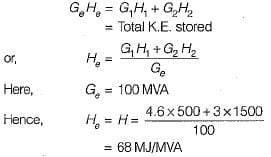Test: Power System Stability of Power Systems - Question 2

### The load carrying capability of a long ac line is

Test: Power System Stability of Power Systems - Question 3

### Fast acting excitation systems

Detailed Solution for Test: Power System Stability of Power Systems - Question 3

Fast field excitation system is one of the method to improve transient stability of the system.

Test: Power System Stability of Power Systems - Question 4

A shunt reactor in general

Detailed Solution for Test: Power System Stability of Power Systems - Question 4

Shunt reactors are used to reduce the effect of Ferranti effect when a medium or a long line is lightly loaded or is on no-load. It doesn’t affect the steady state power transfer limit.

Test: Power System Stability of Power Systems - Question 5

The angle swings during transients

Test: Power System Stability of Power Systems - Question 6

If the inertia constant H = 9.0 MJ/MVA for a 50 MVA generator, the stored energy is

Detailed Solution for Test: Power System Stability of Power Systems - Question 6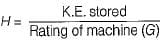or,
K.E. stored = GH = 50 x 9 = 450 MJ

Test: Power System Stability of Power Systems - Question 7

Bundled conductors in a transmission line will

Detailed Solution for Test: Power System Stability of Power Systems - Question 7

Bundled conductors increases self GMD due to which the series inductive reactance is reduced as a result of which power transferred from sending end to the receiving end is increased. Hence, it improves the steady state stability of the system.

Test: Power System Stability of Power Systems - Question 8

A 1500 MVA synchronous generator has an inertia constant of 5.0 MJ/MVA. The inertia constant of the machine relative to a 50 MVA base is

Detailed Solution for Test: Power System Stability of Power Systems - Question 8

Since inertia constant,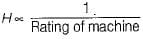∴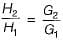or,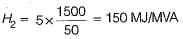Test: Power System Stability of Power Systems - Question 9

If the initial acceleration power of a 100 MVA, 50 Hz synchronous generator having H= 5 MJ/MVA is Xpu, the initial acceleration in elec-deg/s2 and the inertia constant in M J-s/ele-deg respectively will be

Detailed Solution for Test: Power System Stability of Power Systems - Question 9

From swing equation,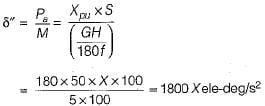Also, inertia constant,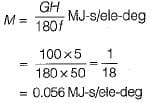Test: Power System Stability of Power Systems - Question 10

The critical clearing angle of a power system is related to

Detailed Solution for Test: Power System Stability of Power Systems - Question 10

tcr and δcr are related to transient stability limit. The fault in a power system must be cleared before δ reaches δcr and before tcr.

## GATE Electrical Engineering (EE) 2023 Mock Test Series

22 docs|274 tests
Information about Test: Power System Stability of Power Systems Page
In this test you can find the Exam questions for Test: Power System Stability of Power Systems solved & explained in the simplest way possible. Besides giving Questions and answers for Test: Power System Stability of Power Systems, EduRev gives you an ample number of Online tests for practice

## GATE Electrical Engineering (EE) 2023 Mock Test Series

22 docs|274 tests(Scan QR code)